# ISEE Middle Level Quantitative : How to find percentage

## Example Questions

← Previous 1 3

### Example Question #1 : How To Find Percentage

Using the information given in each question, compare the quantity in Column A to the quantity in Column B.

Jack scores a 40 on his first test and improves his score by 80% on his second test. Jill scores 50 on her first test, and her second test is 130% of her first test.

Column A          Column B

Jack's 2nd         Jill's 2nd

test score          test score

The relationship cannot be determined from the information given.

The quantity in Column A is greater.

The quantity in Column B is greater.

The two quantities are equal.

The quantity in Column A is greater.

Explanation:

Jack improves by 80%, so we multiply his original score by 1.8 (the 1 to represent his earlier score and the .8 to add on his improvement). 40% times 1.8 equals 72%.

Jill's second test is 130% of her first test (that is, a 30% improvement). To find her new score we multiply her first score by 1.3. 50% times 1.3 equals 65%.

Thus, Jack's second test score is higher.

### Example Question #1 : How To Find Percentage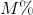of 2,001 is 1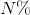of 1,999 is 1

Which is the greater quantity?

(a)(b)(a) and (b) are equal

It is impossible to tell from the information given

(b) is greater

(a) is greater

(b) is greater

Explanation:

No calculation is necessary. The whole in (b) is less, so 1 is a greater portion of that whole than the whole in (a). This makes (b) greater.

### Example Question #3 : How To Find Percentage

Which is the greater quantity?

(a)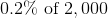(b)(b) is greater

(a) and (b) are equal

(a) is greater

It is impossible to tell from the information given

(a) is greater

Explanation:can be rewritten as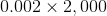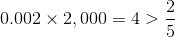### Example Question #4 : How To Find Percentage

1,111 isof 999

999 isof 1,111

Which is the greater quantity?

(a)(b)(a) and (b) are equal

(a) is greater

It is impossible to tell from the information given

(b) is greater

(a) is greater

Explanation:

No calculation is necessary. In (a), the part is greater than the whole, so the percentmust be greater than 100. In (b) The part is less than the whole, somust be less than 100. Therefore,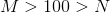### Example Question #2 : How To Find Percentage

There were 48 pieces of fruit brought to the brunch. Twelve of the pieces of fruit were bananas. What percentage of the fruit were bananas?

66%

48%

12%

25%

50%

25%

Explanation:

Percentage involves part over whole. The total number of fruit was 48. The number of bananas was 12. Therefore, you can make a fraction from those numbers: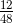. Then, to find the percentage, divide 12 by 48. This gives you 0.25. To find the percentage, move the decimal point to the right two places. This gives you 25%.

### Example Question #6 : How To Find Percentage

70% of 4,000 is equal to what percent of 6,400?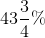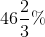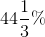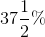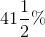Explanation:

70% of 4,000 is equal to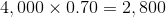, which is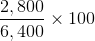percent of 6,400.

Evaluate: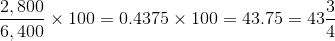The correct response is### Example Question #7 : How To Find Percentageis a positive number. Which of the following is the greater quantity?

(A) 70% of 40% of(B) 40% of 70% of(A) and (B) are equal

(A) is greater

(B) is greater

It is impossible to determine which is greater from the information given

(A) and (B) are equal

Explanation:

40% of a number is the number multplied by 0.40; 70% of the number is the number multiplied by 0.70.

40% ofis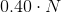; 70% of that is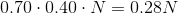70% ofis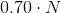; 40% of that is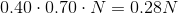Regardless of the value of, the quantities are equal.

### Example Question #8 : How To Find Percentageis a positive integer. Which is the greater quantity?

(A) 25% of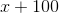(B) 50% of(A) is greater

(A) and (B) are equal

(B) is greater

It is impossible to determine which is greater from the information given

It is impossible to determine which is greater from the information given

Explanation:

The greater of the two can be shown to depend on the value of.

Example 1: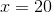Then 25% ofis equal to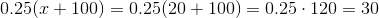and 50% ofis equal to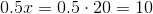This makes (A) greater.

Example 2: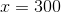Then 25% ofis equal to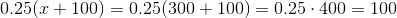and 50% ofis equal to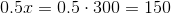This makes (B) greater.

Therefore, insufficient information is given in the problem to determine which is the greater.

### Example Question #9 : How To Find Percentageis a positive number. Which is greater?

(A) 60% of 40% of(B) 50% of 50% ofIt is impossible to determine which is greater from the information given

(A) and (B) are equal

(A) is greater

(B) is greater

(B) is greater

Explanation:

40% ofis equal to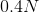; 60% of that is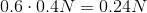50% ofis equal to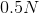; 50% of that is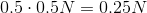Sinceis positive and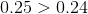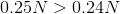,

and (B) is greater.

### Example Question #6 : How To Find Percentage

What is 0.5% of 1,000,000?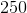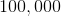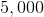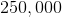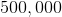Explanation:

Convert the percentage to a decimal: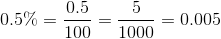Multiply by 1,000,000 to get our answer.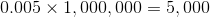← Previous 1 3